Open in App
Not now

# Class 8 RD Sharma Solutions – Chapter 27 Introduction to Graphs – Exercise 27.2

• Last Updated : 30 Apr, 2021

### Question 1. The following table shows the number of patients discharged from a hospital with HIV diagnosis in different years:

Represent the information by a graph.

Solution:

From the question as we know that,

The number of patients – is a dependent variable.

Year – is an independent variable.

Take years on x-axis

and Number of patients on y – axis

Choose the following scale:

X – axis: 2 cm = 1 year

Y – axis: 1 cm = 10 patients

Consider that on the x-axis, origin (O) represents 2001 and on the y-axis, origin (O) represents 120,

i.e. O (2001, 120).

Now, Plot

(2002, 150),

(2003, 170),

(2004, 195),

(2005, 225),

(2006, 230).

Join these points in the following graph as shown below.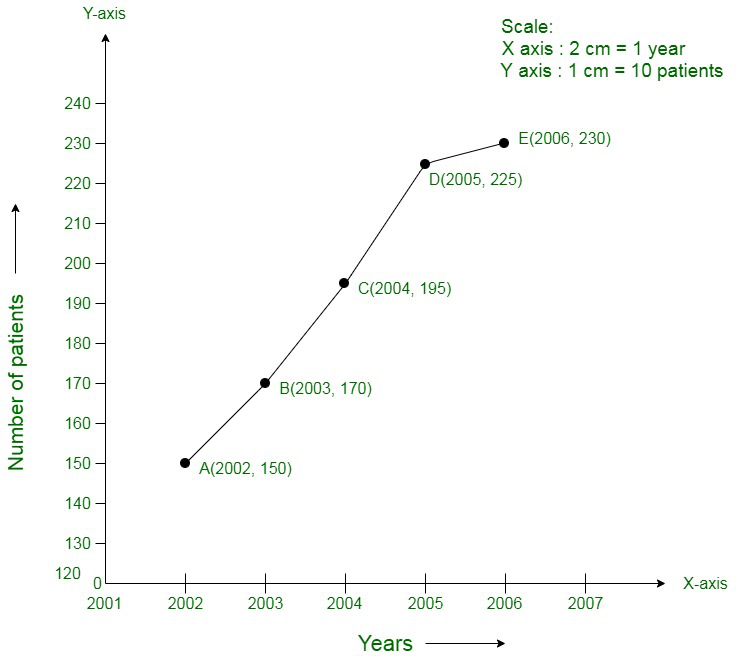### Question 2. The following table shows the amount of rice grown by a farmer in different years:

Plot a graph to illustrate this information.

Solution:

From the question as we know that,

Year – is an independent variable

Quantity of rice grown is a dependent variable.

So, take

Year on X – axis

Quantity of rice grown on Y – axis

Choose following scale:

X – axis: 2 cm = 1 year

Y – axis: 1 cm = 20 quintals

Consider that the origin O represents the coordinates (1999, 160).

i.e. O (1999,160)

Now, Plot

(2000, 200),

(2001, 180),

(2002, 240),

(2003, 260),

(2004, 250),

(2005, 200),

(2006, 270).

Join these points on the following graph as shown below: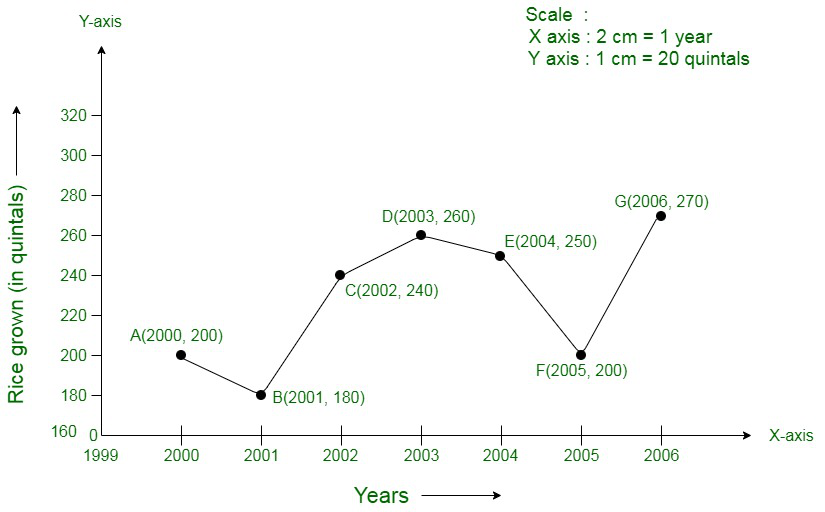### Question 3.The following table gives the information regarding the number of persons employed to a piece of work and time taken to complete the work:

Plot the graph of this information.

Solution:

From the question as we know that,

The number of persons – is an independent variable

Time taken – is a dependent variable.

So, take

The number of persons on the X – axis

Time taken on the Y – axis.

Choose following scale:

X – axis: 2 cm = 2 persons

Y – axis: 2 cm = 2 days

Now, plot

(2, 12),

(4, 6),

(6, 4),

(8, 3).

Join these points on the following graph as shown below: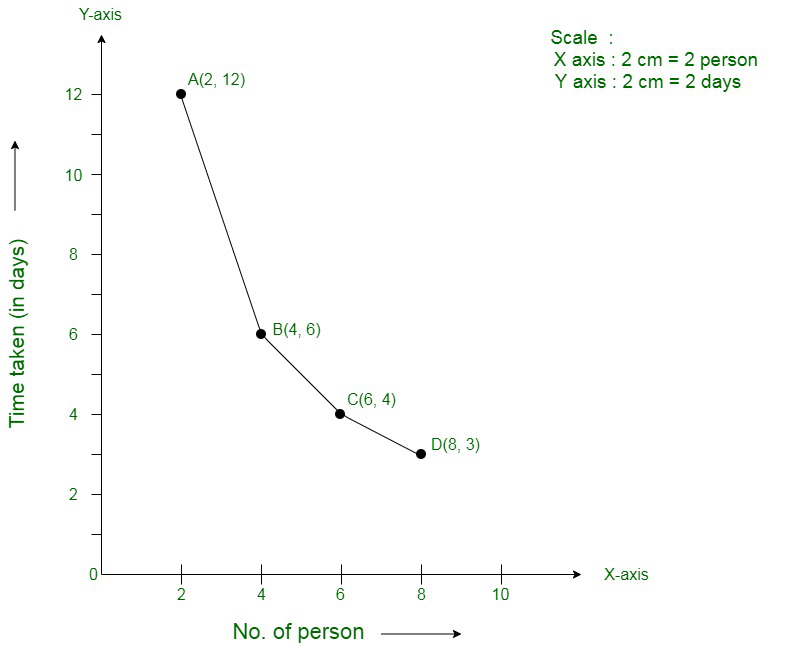### Question 4. The following table gives the information regarding length of a side of a square and its area

Draw a graph to illustrate this information.

Solution:

From the question as we know that,

Length of a side – is an independent variable

Area of the square – is a dependent variable.

So, take

Length of a side on the X – axis

Area of the square on the Y – axis.

Choose following scale:

X – axis: 2 cm = 1 cm

Y – axis: 1 cm = 2 cm2

Now, plot

(1, 1),

(2, 4),

(3, 9),

(4, 16),

(5, 25).

Join these points in the following graph as shown below: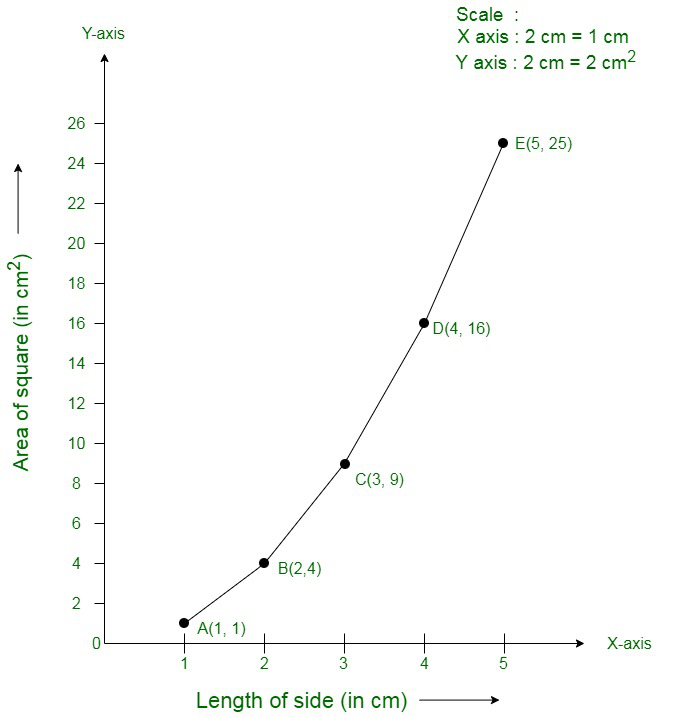### Question 5. The following table shows the sales of a commodity during the years 2000 to 2006.

Draw a graph of this information.

Solution:

From the question as we know that,

Year – is an independent variable

Sales – is a dependent variable.

So, take

Year on the X – axis

Sales on the Y – axis.

Choose following scale:

X – axis: 2 cm = 1 year

Y – axis: 2 cm = 1 lakh rupees

Consider that on x-axis, origin (O) represents 1991.

Hence, the coordinates of O are (1991, 0).

Now, plot

(2000, 1.5),

(2001, 1.8),

(2002, 2.4),

(2003, 3.2),

(2004, 5.4),

(2005, 7.8)

(2006, 8.6).

Join these points on the following graph as shown below;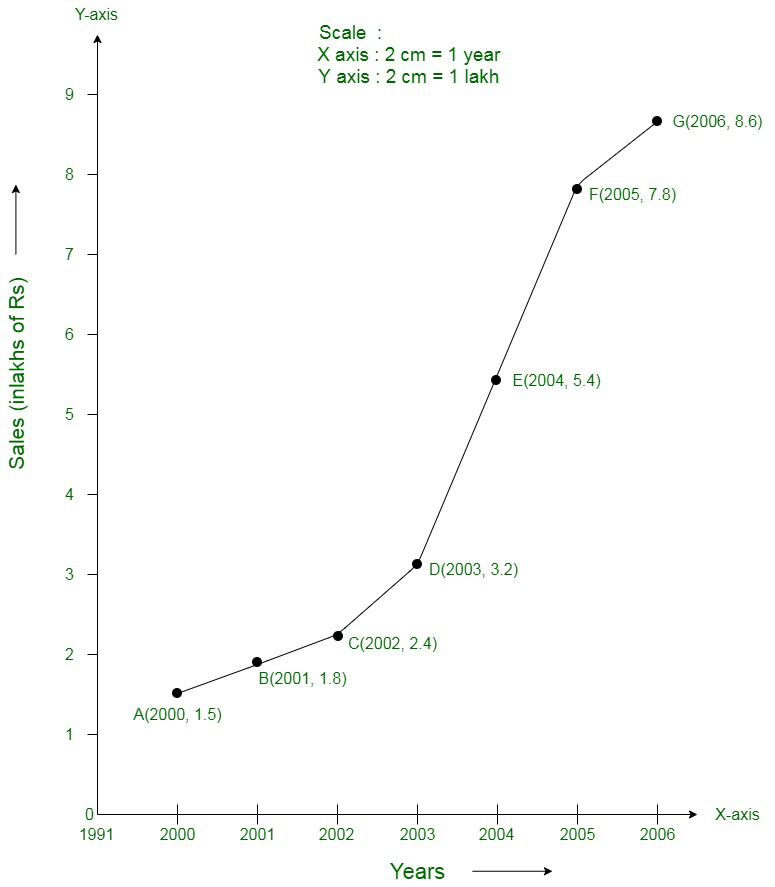### Question 6. Draw the temperature-time graph in each of the following cases:

(i)

(ii)

Solution:

(i) From the question as we know that,

Time – is an independent variable

Temperature – is a dependent variable.

So, take

Time on the X – axis

Temperature on the Y – axis.

Choose following scale:

X – axis: 1 cm = 1 hour

Y – axis: 1 cm = 1°F

Consider that on the x-axis, the coordinate of origin is 6:00.

On y-axis, the coordinate of origin is 94°F.

Hence, the coordinates of 0 are (6:00, 94).

Now, plot

(7:00, 100),

(9:00, 101),

(11:00, 104)

(21:00, 98)

Join these points on the following graph as shown below: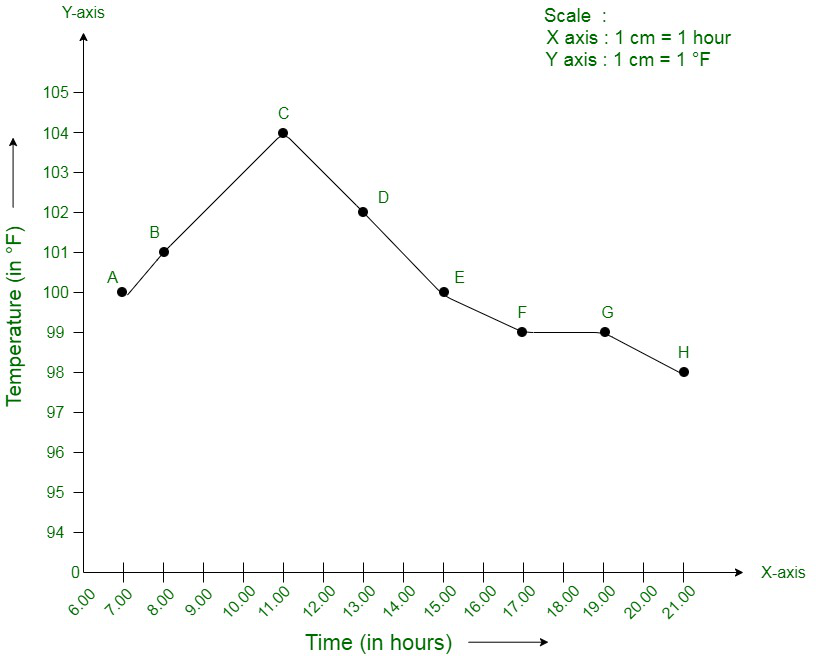(ii) From the question we know that,

Time – is an independent variable

Temperature – is a dependent variable.

So, take

Time on the X – axis

Temperature on the Y – axis.

Choose following scale:

X – axis: 2 cm = 2 hours

Y – axis: 1 cm = 1°F

Consider that on the x-axis, the coordinate of origin is 6:00.

On y-axis, the coordinate of origin is 94°F.

Thus, the coordinates of 0 are (6:00, 94).

Now, plot

(8:00, 100),

(10:00, 101),

(12:00, 104)…..

(20:00, 100).

Join these points on the graph as shown below: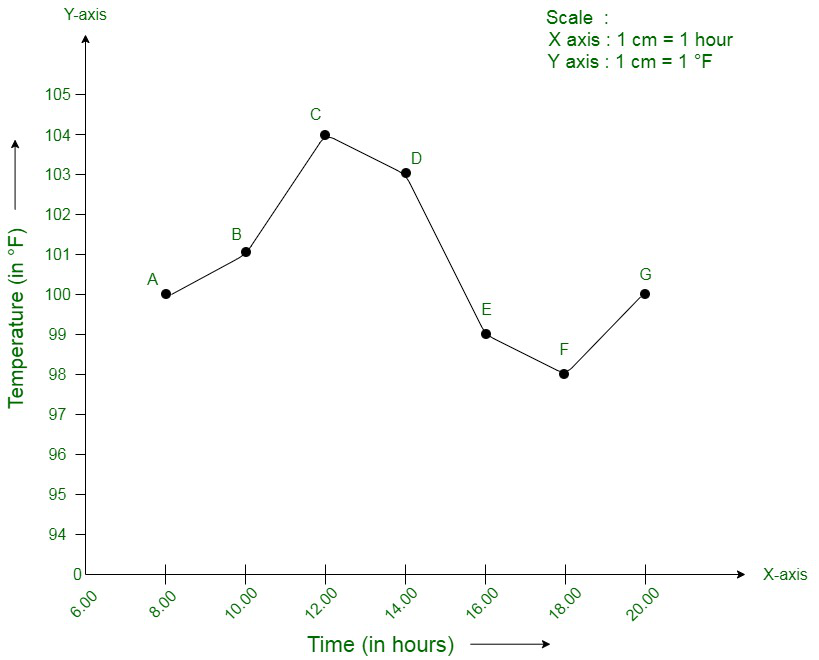### Question 7. Draw the velocity-time graph from the following data:

Solution:

From the question as we know that,

Time – is an independent variable.

Speed – is a dependent variable.

So, take

Time on the X – axis

Speed on the Y – axis.

Choose following scale:

X – axis: 2 big division = 1 hour

Y – axis: 1 big division = 10 km/hr

Consider that on the x-axis, the coordinate of origin (O) is 7:00.

Thus, the coordinates of O are (7:00, 0).

Now, plot

(7:00, 30),

(8:00, 45),

(9:00, 60),

(10:00, 50),

(11:00, 70),

(12:00, 50),

(13:00, 40),

(14:00, 45).

Join these points on the graph as shown below: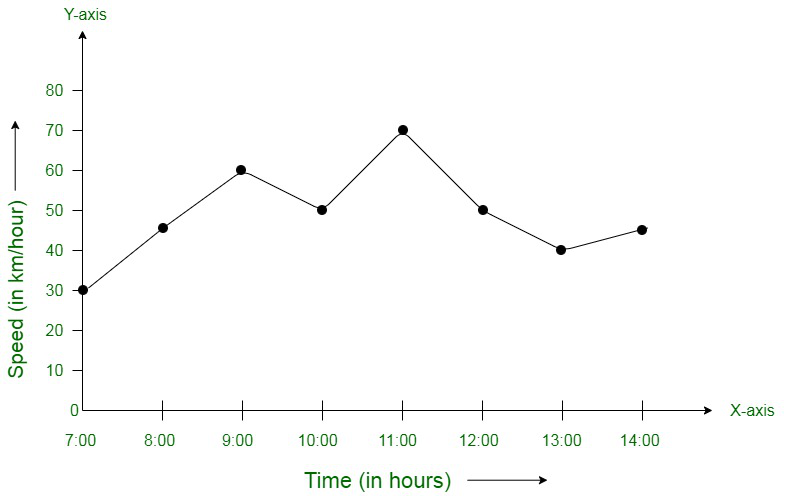### Draw the graph representing the above data in two different ways as a graph and as a bar chart.

Solution:

From the question as we know that,

Over – is an independent variable.

Run is a dependent variable.

So, take

Overs on the X – axis

Runs on the Y – axis.

Choose following scale:

X – axis: 1 cm = 1 over

Y – axis: 1 cm = 2 runs

Now, plot

(I, 2),

(II, 1),

(III, 4)…

(XV, 12).

Join these points on graph as shown below: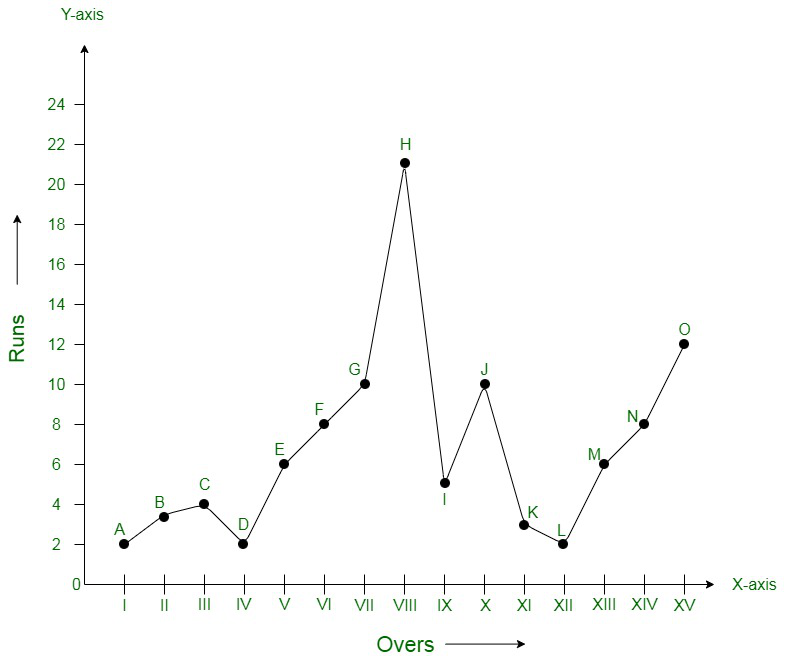The bar graph is: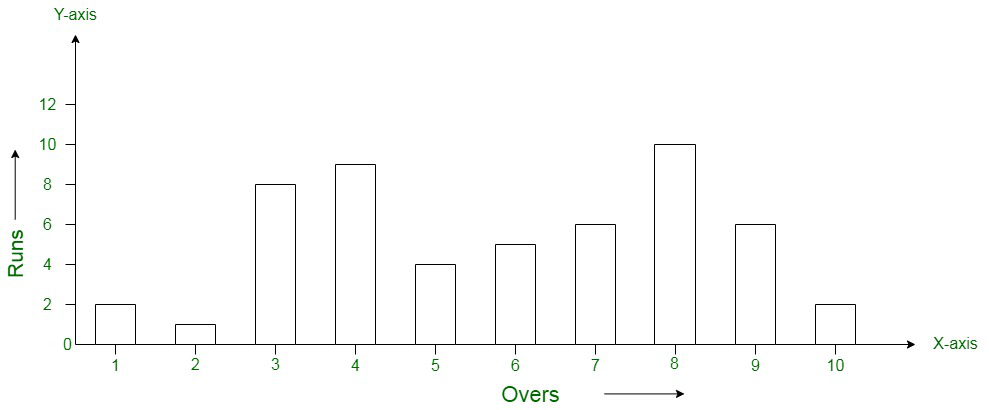### Draw a graph depicting the data, making the graphs on the same axes in each case in two different ways as a graph and as a bar chart.

Solution:

From the question as we know that,

Over – is an independent variable.

Run – is a dependent variable.

So, take

Overs on X – axis

Runs on the Y – axis.

Choose following scale:

X – axis: 1 cm = 1 over

Y – axis: 1 cm = 1 run

Now, plot

(I, 2),

(II, 1),

(III, 8)…

(X, 2) for team A

and

(I, 5),

(II, 6),

(III, 8) …..

(X, 10) for team B.

Join these points on graph as shown below: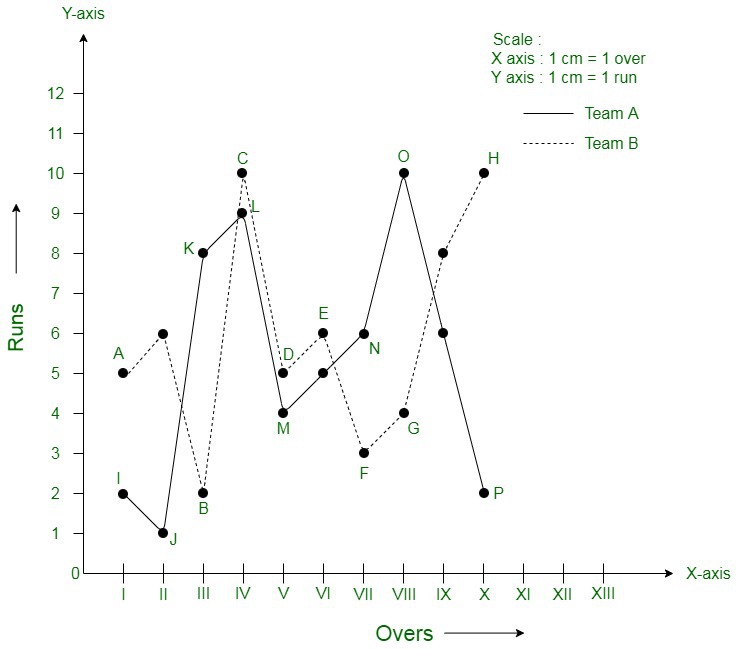The bar graph is: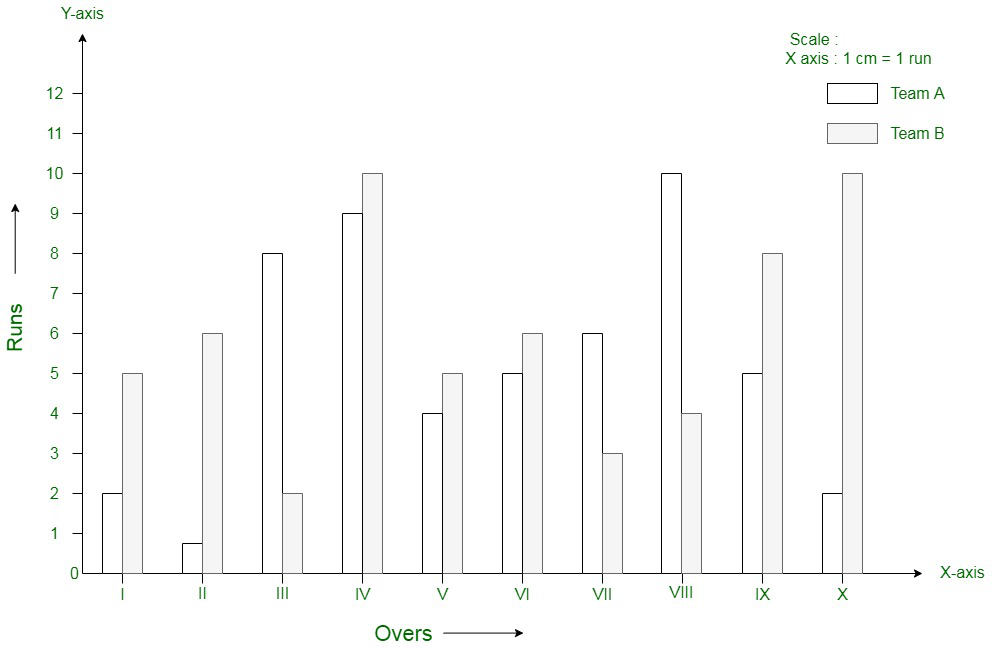My Personal Notes arrow_drop_up